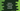# Python math trunc method explanation with example## Python math trunc() method:

The trunc() method is defined in the python math module. This is used to truncate a number in python. It truncates the decimal part of a number and returns the integer part. We can provide a positive or negative value to the trunc() method.

For a positive value, it is similar to floor() and for a negative value, it is similar to ceil().

### Syntax of math.trunc():

math.trunc() method is defined as like below:

``math.trunc(num)``

Here, num is the number we are truncating. It will return the truncated value.

It returns the truncate value of the number num.

### Errors:

math.trunc() might throw OverflowError or ValueError. It will throw an OverflowError if the value of the number num is infinity. Similarly, it will throw a ValueError if the number num is NaN.

### Example of math.trunc with positive numbers:

Let’s try math.trunc with positive values:

``````import math

arr = [1, 0, 1.34, 1.36, 1.39999, 1.22309989, 232]

for item in arr:
print(f'math.trunc({item}) = {math.trunc(item)}')``````

We are using math.trunc with different positive numbers of arr. It will print:

``````math.trunc(1) = 1
math.trunc(0) = 0
math.trunc(1.34) = 1
math.trunc(1.36) = 1
math.trunc(1.39999) = 1
math.trunc(1.22309989) = 1
math.trunc(232) = 232``````

### Example of math.trunc with negative numbers:

Let’s try math.trunc with negative numbers.

``````import math

arr = [-1, -0, -1.34, -1.36, -1.39999, -1.22309989, -232]

for item in arr:
print(f'math.trunc({item}) = {math.trunc(item)}')``````

### Example of math.trunc with a string, TypeError:

If you try to use math.trunc with a string variable, it will throw one error:

``````import math

str = '20'

print(f'math.trunc({str}) = {math.trunc(str)}')``````

It will print:

``````Traceback (most recent call last):
File "example.py", line 5, in <module>
print(f'math.trunc({str}) = {math.trunc(str)}')
TypeError: type str doesn't define __trunc__ method``````

We can’t use trunc with a string.

#### How to use trunc with valid string numbers:

As you have seen above, we can’t use trunc directly with a string, but we can always convert it to a float with the float() method and use math.trunc on the result:

``````import math

str = '20.334'

print(f'math.trunc({str}) = {math.trunc(float(str))}')``````

It will give:

``math.trunc(20.334) = 20``

#### math.trunc with a list of values:

In most cases, you will get the values in a list. If you want to truncate all items of a list, you can’t do it directly. If you try to use math.trunc on a list, it will throw TypeError. Instead, we can use a loop to loop through each item of the list and truncate each individually.

``````import math

nums = [1, 2.34, -9980.484, 5.98, -9.98, 66.575, -1]

truncate_nums = [math.trunc(n) for n in nums]

print(f'math.trunc({nums}): {truncate_nums}')``````

Output:

``math.trunc([1, 2.34, -9980.484, 5.98, -9.98, 66.575, -1]): [1, 2, -9980, 5, -9, 66, -1]``

#### math.trunc with a tuple of values:

Similar to a list, we can also use math.trunc with a tuple of values.

``````import math

nums = (1, 2.34, -9980.484, 5.98, -9.98, 66.575, -1)

truncate_nums = [math.trunc(n) for n in nums]

print(f'math.trunc({nums}): {truncate_nums}')``````

It will give the same output.

#### OverflowError in math.trunc:

It throws OverflowError if we pass infinity as the parameter.

``````import math

print(math.trunc(math.inf))``````

Output:

``````Traceback (most recent call last):
File "example.py", line 3, in <module>
print(math.trunc(math.inf))
OverflowError: cannot convert float infinity to integer``````

#### ValueError in math.trunc:

ValueError is thrown if you pass NaN as the parameter:

``````import math

print(math.trunc(math.nan))``````

Output:

``````Traceback (most recent call last):
File "example.py", line 3, in <module>
print(math.trunc(math.nan))
ValueError: cannot convert float NaN to integer``````

#### TypeError:

For any invalid value, it throws TypeError:

``````import math

print(math.trunc('xx'))``````

Output:

``````Traceback (most recent call last):
File "example.py", line 3, in <module>
print(math.trunc('xx'))
TypeError: type str doesn't define __trunc__ method``````